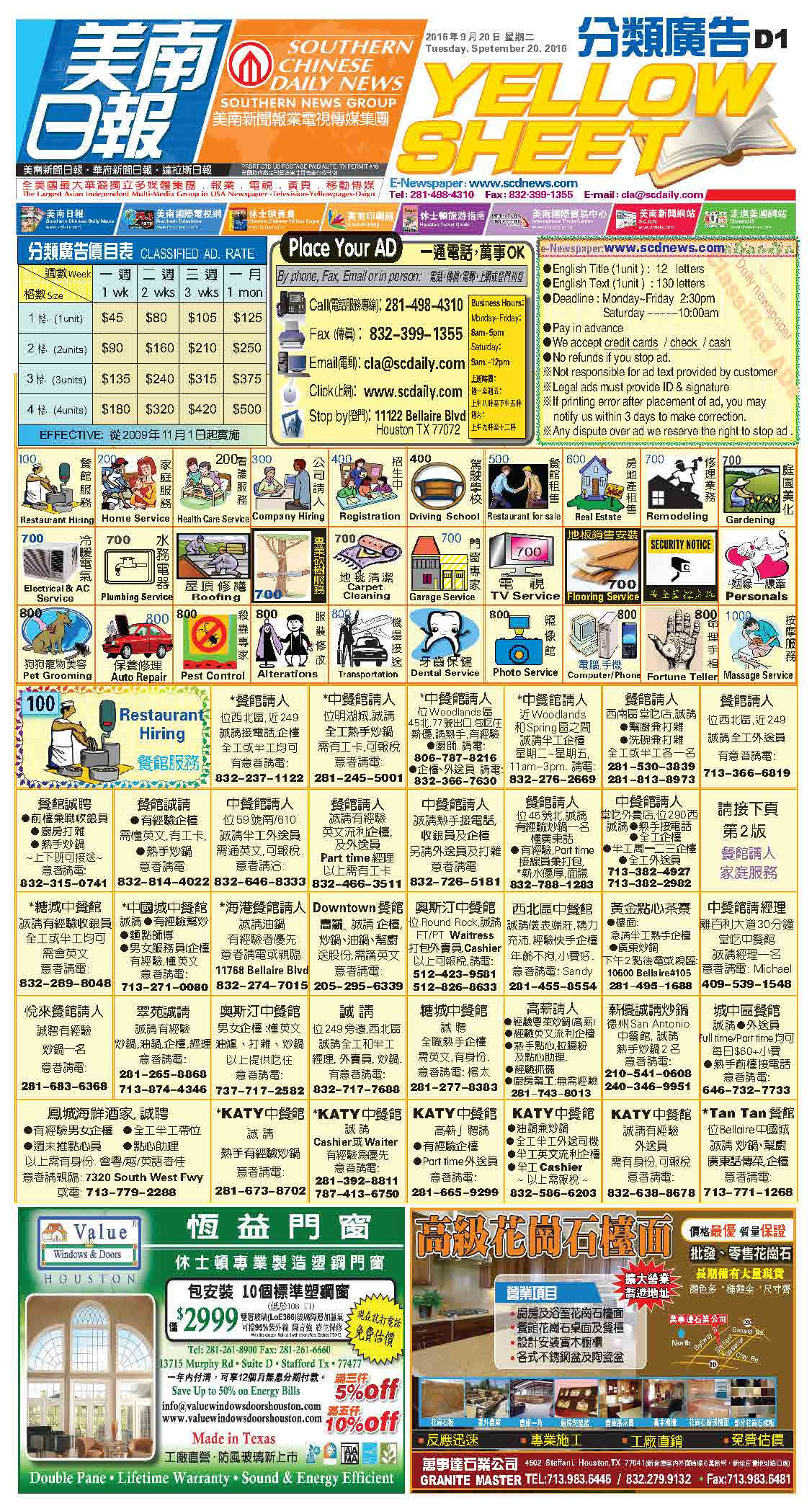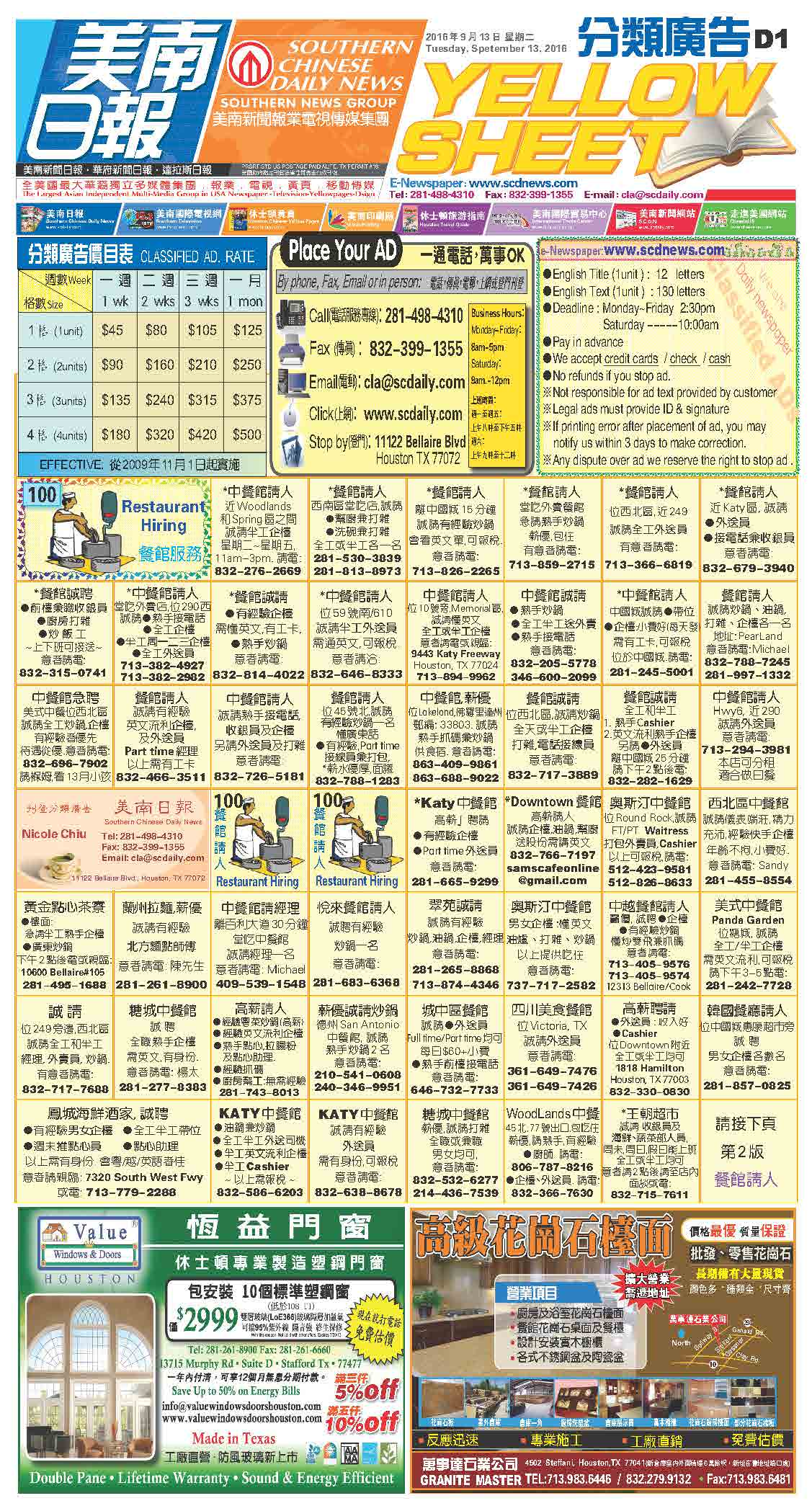160923 Epaper

 A Section B SectionC Section D Section160922 Epaper

 A Section B SectionC Section D Section160921 Epaper

 A Section B SectionC Section D Section160920 Epaper

 A Section B SectionC Section D Section160919 Epaper

 A Section B SectionC Section D Section160918 Epaper

 A Section B SectionC Section D Section160917 Epaper

 A Section B SectionC Section D Section160916 Epaper

 A Section B SectionC Section D Section160914 Epaper

 A Section B SectionC Section D Section160915 Epaper

 A Section B SectionC Section D Section160913 Epaper

 A Section B SectionC Section D Section